﻿ Dual Reciprocity Boundary Element Method for Steady State Convection-Diffusion-Radiation problems

### Dual Reciprocity Boundary Element Method for Steady State Convection-Diffusion-Radiation problems

Hassan Zakerdoost, Hassan GhassemiOPEN ACCESSPEER-REVIEWED

## Dual Reciprocity Boundary Element Method for Steady State Convection-Diffusion-Radiation problems

Hassan Zakerdoost1,, Hassan Ghassemi1

1Department of Ocean Engineering, Amirkabir University of Technology, Tehran, Iran

### Abstract

Many problems in fluid dynamics and heat transfer are defined by nonlinear equations. In this paper steady state convection-diffusion-reaction (SSCDR) equations are solved by dual reciprocity boundary element method (DRBEM). DRBEM is employed to transform the domain integrals into the boundary only integrals by employing the fundamental solution of the Laplace Equation. The linear Radial Basis Functions (RBFs) is used in the dual reciprocity technique. To verify the accuracy of the approach, the numerical results of an examples is calculated and compared with the analytical solution. The comparison demonstrates the usefulness of the approach for diffusion-dominated problems with low velocity values.

12
Prev Next

### Cite this article:

• Zakerdoost, Hassan, and Hassan Ghassemi. "Dual Reciprocity Boundary Element Method for Steady State Convection-Diffusion-Radiation problems." International Journal of Partial Differential Equations and Applications 2.4 (2014): 68-71.
• Zakerdoost, H. , & Ghassemi, H. (2014). Dual Reciprocity Boundary Element Method for Steady State Convection-Diffusion-Radiation problems. International Journal of Partial Differential Equations and Applications, 2(4), 68-71.
• Zakerdoost, Hassan, and Hassan Ghassemi. "Dual Reciprocity Boundary Element Method for Steady State Convection-Diffusion-Radiation problems." International Journal of Partial Differential Equations and Applications 2, no. 4 (2014): 68-71.

 Import into BibTeX Import into EndNote Import into RefMan Import into RefWorks

### 1. Introduction

The two dimensional (2D) steady state convection-diffusion-reaction (SSCDR) discussed in this paper is combining of 2D steady state convection equation and 2D steady state diffusion equation with a linear reaction term. These equations and their combinations can be governed many transport problems in fluid dynamics [1, 2, 3]. Modeling chemical, physical, environmental and biological phenomena mathematically are samples of these transport problems.

Some researchers used different methods for solving partial differential equations (PDEs) [4, 5, 6]. The presence of the first-order derivatives in the CDR equations causes skew-symmetric coefficient matrices with potentially destabilizing effects. Thus some authors attempted to solve this problem by different numerical schemes but today we are still required better numerical technique for more accurate approximating PDEs that include first order spatial derivatives (convective term) and hence it is an attractive and challenging issue in fluid dynamics problems .

The DRBEM which was originally introduced by Nardini and Brebbia , is thus the most successful method since it reduces the dimension of the problem by one unit and then saves computational time and memory. The DRBEM rearranges the PDE terms into equations of the formsand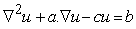(the coefficientsand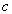are constants), that have the fundamental solutions and then approximates the non-homogeneous termby interpolating functions, called radial basis functions (RBFs) in terms of its values at the collocation. Dehghan and Mirzaei  have applied the boundary integral equation and DRBEM for solving 1D Cahn–Hilliard equation. Yun and Ang  treated the DRBEM for axisymmetric thermo-elastostatic analysis of non-homogeneous materials. In  the dual reciprocity boundary integral equation technique has been presented to solve the nonlinear Klein–Gordon equation. Purbolaksono and Aliabadi employed the DRBEM to evaluate large deformations of shear deformable plates . Recently, Damapack et al applied boundary element method to the bending analysis of thin functionally graded plates .

In the present paper, the well-known DRBEM is applied to numerically solve the 2D steady state convection-diffusion-reaction problems. The outline of this work is as follows: In Section 2, the governing equation and boundary condition of problem are presented. The discretization of the steady state convection-diffusion-reaction equation using dual reciprocity boundary element method is described in Section 3. The numerical and analytical results are compared in Section 4. And, last section summarizes conclusions of the numerical results.

### 2. Problem Statement

Consider a temperature field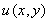in a homogeneous isotropic domain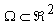bounded by a piece-wise smooth boundary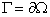such that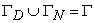andwith Dirichlet and Neumann boundary conditions respectively. The mathematical equation governing steady state convection-diffusion-reaction type problems in 2D is:(1)

With respect to a particular problem, u can demonstrate a temperature or the concentration of a chemical species. D > 0 is the diffusion coefficient of the temperature or the species,is the velocity field of fluid flow and k > 0 is a reaction coefficient.

Boundary conditions of the problem are of the form: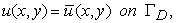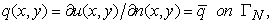(2)

where n is the outward normal vector to the boundary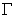,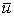andare known boundary functions.

### 3. DRBEM Formulation

There are several analytical and numerical methods for solving the above steady state boundary value problem. We employ boundary integral equation and the DRBEM for Equation (1) subject to boundary conditions (2) to find the BEM solution. Because the velocity field may be variable and the fundamental solution of the Laplace operator is available, the convective and reaction terms are shifted to the right hand side (RHS) and then the Equation (1) can be rewritten as:(3)

Now to expand the non-homogeneity of the above equation (i.e. function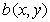) in terms of its values at each nodes based on the idea of the DRBEM, we utilize a set of RBFs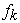such that(4)

where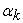a set of undetermined interpolation coefficients, N is the number of collocation nodes on the boundary, L is the number of collocation points in the domain and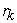is defined as the distance between the node under consideration and the node k.

In [14, 15, 16], authors found that the following RBF is effective and has good results with most of problems.(5)

The vectoris obtained from Equation (4) as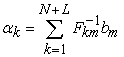(6)

Where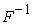is the inverse of matrix f. we can find a particular solution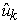associated with each functionsatisfying the following equation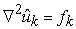(7)

Substituting Equation (8) into Equation (4), we have(8)

Let us consider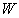as the weighting function and the fundamental solution of Laplace equation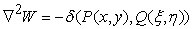,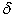is the Dirac delta function and P and Q are a field point and a source point respectively. The fundamental solution or weighting function is given as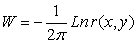(9)

As defined previous in Equation (3)is a function of the first order derivative of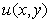. We can utilize a global interpolation function forto approximate the values of its derivatives at all the collocation points in similar way to that of for. We choose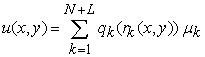(10)

where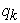is the RBF that is appropriate and compatible for the problems contained derivatives in the functionand it is defined as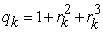(11)

and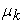are unknown coefficients for each collocation point that is determined as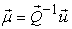(12)

where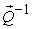is the inverse of matrix q. Applying the weighted residual technique to Equation (3) and after some matrix manipulation (for more details see ) we have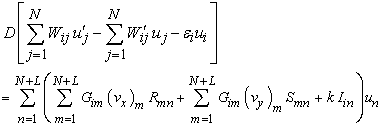(13)

For the constant element case, where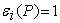for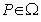and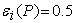for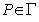, the prime symbol denotes the normal derivative of the functions,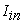is the Identity matrix and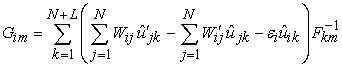(14)

The Equation (13) is the discretized version of the Poisson equation using DRBEM and the problem has been reduced from a mixed formulation containing boundary integrals and a domain integral to a boundary only formulation [11, 19]. The source point i is moved to all N boundary and L DRM nodes on the boundary and in the interior to develop N+L linear equation in N+L unknowns and to close the problem. Introducing the boundary conditions (2) into Equation (13) and rearranging by taking known variables to the right hand side, and unknown variables to the left hand side, we can get a linear algebra system of equations that may be solved by employing the usual Gauss elimination method or the efficient LU decomposition technique.

### 4. Numerical Results

This example is a two-dimensional SSCDR problem on the square domainthat the convective coefficient is a function of the second order in direction y coordinate. Here, 200 boundary nodes and 513 internal nodes have been used. The code of the method procedure is written in DVF-FORTRAN 95. The convective coefficient is a second order function of y-coordinate. The Equation is given by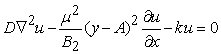(15)

whereand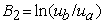. Here, the value of parameter A is assumed by two values as A=0.5 and A=0.25 to make respectively the profile of the convective coefficient and the functionsymmetric and non-symmetric with respect to the y-coordinate. The boundary conditions correspond to the problems are defined as(16)

A particular solution of the above equation can be obtained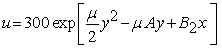(17)

Figure 1 and Figure 2 show the comparison between the obtained horizontalprofile and the corresponding analytical solution along the faces y=0 and y=1 for A=0.5 and A=0.25 respectively. Figure 3 and Figure 4 present relative errors of the normal derivative (|(numerical − analytical)/analytical|). With respect to the accuracy achieved, it can be seen from these figures good numerical results for the normal derivative have been obtained along the vertical boundary x=0 and x=1. The differences between the analytical solution and the numerical solution for several horizontal lines of the domain can be demonstrated from Figure 5 and Figure 6 for A=0.5 and A=0.25 respectively. These both figures indicate that as the term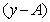limits to zero the relative errors decrease to zero.

Figure 1. Comparison of numerical and analytical solutions for the function u(x) along horizontal boundaries, y=0, y=1 for A=0.50
Figure 2. Comparison of numerical and analytical solutions for the function u(x) along horizontal boundaries y=0, y=1 for A=0.25
Figure 3. Relative errors between numerical and analytical solutions for the normal derivate along the vertical boundary x=1 for A=0.50 and A=0.25
Figure 4. Relative errors between Numerical and analytical solutions for the normal derivate along the vertical boundary x=0 for A=0.50 and A=0.25
Figure 6. Relative errors of function u(x,y) for five horizontal faces for A=0.25

### 5. Conclusions

In this study the formulation of the boundary integral Equation and the dual reciprocity boundary element method were employed for solving the SSCDR equation on two-dimensional domain. Discretized form of the Equation was obtained by using the proposed method and its procedure was described in detail. Moreover, the significant advantage and robustness of the DRBEM permitted us to implement it for SSCDR transport problems with variable convective coefficient. The accuracy and efficiency of this technique were evaluated and good agreement between numerical results and the corresponding analytical solutions was achieved.

### References

  Man, C. and Tsai, C.W., “A higher-order predictor-corrector scheme for two-dimensional advection-diffusion Equation,” Int. J. Numer. Meth. Fluids, 56 (4), 401-418. 2007.In article CrossRef  Phongthanapanich, S. and Dechaumphai, P., “Combined finite volume and finite element method for convection-diffusion-reaction Equation,” Journal of Mechanical Science and Technology, 23, 790-801. 2009.In article CrossRef  Li, Y. and Yin, L., “A New Surfactant Flooding Model for Low Permeability Reservoirs,” Open Journal of Fluid Dynamics, 3 (1), 1-8. 2013.In article CrossRef  Gomez, H., Colominas, I., Navarrina, F. and Casteleiro, M., “A discontinuous Galerkin method for a hyperbolic model for convection-diffusion problems in CFD,” Int. J. Numer. Meth. Eng., 71 (11), 1342-1364. 2007.In article CrossRef  Rashidi, M.M., Domairry, G., Dinarvand, S., “Approximate solutions for the Burger and regularized long wave equations by means of the homotopy analysis method,” Comm Nonlinear Sci Numer Simulat, 14 (3), 708-717. 2007.In article CrossRef  Rashidi, M.M., Ganji, D.D., Dinarvand, S., “Explicit analytical solutions of the generalized Burger and Burger–Fisher equations by homotopy perturbation method,” Numerical Methods for Partial Differential Equations, 25 (2), 409-417. 2008.In article CrossRef  Rashidi, M.M., Erfani, E., “New analytical method for solving Burgers' and nonlinear heat transfer equations and comparison with HAM,” Computer Physics Communications, 180 (9), 1539-1544. 2009.In article CrossRef  Nardini, D. and Brebbia, C.A., “A new approach to free vibration analysis using boundary elements,” Appl Math Model, 7, 157-62. 1983.In article CrossRef  Dehghan, M. and Mirzaei, D., “A numerical method based on the boundary integral equation and dual reciprocity methods for one-dimensional Cahn-Hilliard Equation,” Eng Anal Bound Elem., 33, 522-528. 2009.In article CrossRef  Yun, B.I. and Ang, W.T., “A dual-reciprocity boundary element method for axisymmetric thermoelastostatic analysis of non-homogeneous materials,” Eng Anal Bound Elem., 36 (12), 1776-1786. 2012.In article CrossRef  Dehghan, M. and Ghesmati, A., “Application of the dual reciprocity boundary integral Equation technique to solve the nonlinear Klein–Gordon Equation” Computer Physics Communications, 181, 1410-1418. 2010.In article CrossRef  Purbolaksono, J. and Aliabadi, M.H., “Stability of Euler's method for evaluating large deformation of shear deformable plates by dual reciprocity boundary element method,” Eng Anal Bound Elem., 34 (9), 819-823. 2010.In article CrossRef  Damanpack, A.R., Bodaghi, M., Ghassemi, H. and Sayehbani, M., “Boundary element method applied to the bending analysis of thin functionally graded plates,” Latin Amer Journal of Solids and Structure, 12, 549-570. 2013.In article CrossRef  Partridge, P.W., Brebbia, C.A. and Wrobel, L.C., The dual reciprocity boundary element method, Computational Mechanics Publications, Southampton, 1992.In article  Partridge, P.W., “Dual reciprocity BEM: Local versus global approximation functions for diffusion, convection and other problems,” Eng Anal Bound Elem., 14, 349-356. 1994.In article CrossRef  Yamada, T., Wrobel, L.C., Power, H., “On the convergence of the dual reciprocity boundary element method,” Eng Anal Bound Elem., 13, 291-298. 1994.In article CrossRef  Partridge, P.W., and Brebbia, C.A., “Computer implementation of the BEM dual reciprocity method for the solution of general field Equations,” Commun. Appl. Numer. Methods, 6, 83-92. 1990.In article CrossRef  Zhang, Y. and Zhu, S., “On the choice of interpolation functions used in the dual-reciprocity boundary-element method,” Eng Anal Bound Elem., 13, 387-396. 1994.In article CrossRef  Ramachandran, P.A., Boundary Element Methods in Transport Phenomena, Computational Mechanics Publication, Southampton, Boston, 1994.In article